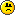SB Physics Help Room Sucks!
Would you like to react to this message? Create an account in a few clicks or log in to continue.

# Maple TA 25_2##Maple TA 25_2

Here's a head start on 25_2

User X
Guest

##Maple TA 25_2

1. A photon has a wavelength of 655.2 nm. What is the photon momentum in eV/c (sheet 14). Indicate with a positive (negative) sign whether the momentum unit quoted is (not) an SI unit.

= (6.64E-34)*(3E8)
=(1.98E-25)
THEN
=(1.98E-25)/(655.2E-9)
=(3.02198E-19)
THEN
=(3.02198E-19)/(1.6E-19)
=1.88874

Reasoning: First step is to get the value for value for (hc) then you divide by the wavelength to get the value of E, and lastly divide by 1.6E-19 to get the value in terms of eV instead of Joules.

User X
Guest

##Maple TA 25_2

BTW the answer for # 1 is NEGATIVE

User X
Guest

##Maple TA 25_2

Still trying to figure out # 2 and # 3

User X
Guest

##Maple TA 25_2

4. An atom makes a transition from its current state into an energy state which is 2.067 eV higher. Indicate with a negative (positive) sign whether a photon is emitted (absorbed) by the atom and calculate the wavelength of the photon (sheet 32).

=(6.64E-34)*(3E8)
=(1.992E-25)
THEN
=(1.992E-25)/(1.6E-19)
=(1.245E-6)
THEN
=(1.245E-6)/(2.067)
=(6.02322E-7)(POSITIVE)

User X
Guest

##Maple TA 25_2

2. A 17.37 KeV photon scatters off an electron (Compton Effect) and has a wavelength of 0.099 nm after the collision. What is the kinetic energy of the recoil electron in KeV (sheet 15-18) ? Indicate with a positive (negative) sign whether the electron kinetic energy is of the same order of magnitude as (considerably smaller than) the electron rest energy.

=((6.64E-34)*(3E8))/(.099E-9)
=(2.01212E-15)
THEN
=(2.01212E-15)/(1.6E-19)
=(12575.8eV)---->12.5758 KeV (in terms of KeV is what the question is asking for)
THEN
=(17.37) - (12.5758)
= (4.7942)(NEGATIVE)

Reasoning: You need to first solve to get "E", and then you divide that answer by 1.6E-19 to convert into eV, and then divide by 1000 to convert from eV to KeV. Then to find the kinetic energy of the recall electron, you subtract the two.

User X
Guest

##Maple TA 25_2

I'm having some trouble with # 3, if anyone can help with that one, please.

User X
Guest

##Maple TA 25_2

Still having no luck with # 3, if anyone can help.

User X
Guest

##question 3

Any ideas on number 3 I cant figure it out either?

sjames
Guest

###3

first divide theta by 2 and convert the d into meters
lambda = (2dsin(theta))/n

second p = h/lambda from above

third KE= (p from above)^2/(2*9.1*10^-31)

forth convert to eV KE from above /(1.6*10^-19)

supr mo
Guest

##Question 3

What is n in the first formula provided?

An electron beam scattered off a crystal at a scattering angle of 26.99 0 produces a second order diffraction maximum. What is the kinetic energy (nonrelativistically) of the electrons in eV if the crystal plane spacing is 0.2637 nm (sheet 22,27) ? Indicate with a positive (negative) sign whether here the particle (wave) property of the electron becomes evident.

what i did so far was 2*.2637E-9= 5.274E10*sin(13.495)/n, but what is n?

Guest786
Guest

hwilson
Guest

##question 3

I am having trouble with that one as well if someone could post a more detailed description of how to solve it I would appreciate it. Thanks.

sck105
Guest

##sck105?

sck105....what do you mean by more details? i thought the details above by userx was very detailed lol.

phyfrk
Guest

##Maple TA 25_2

Here's a full description on question 3.
An electron beam scattered off a crystal at a scattering angle of 27.9 0 produces a second order diffraction maximum. What is the kinetic energy (nonrelativistically) of the electrons in eV if the crystal plane spacing is 0.2926 nm (sheet 22,27) ? Indicate with a positive (negative) sign whether here the particle (wave) property of the electron becomes evident.
=(27.9)/2
=13.95
THEN
=(2)*(.2926E-9)*sin(13.95)
=(4.70665E-24)/2
=7.05386E-11
THEN
=(6.64E-34)/(7.05386E-11)
=9.41329E-29
THEN
=(9.41329E-29)^2
=8.861E-47
THEN
=(8.861E-47)/(2*9.1E-31)
=4.86868E-17
THEN
=(4.86868E-17)/(1.6E-19)
=304.293 (NEGATIVE)

User X
Guest

##3

I am still getting it wrongmy numbers were 34.84 and .15nm
my final answer came to -75.10 but it says wrong
can someone help me:(
Guest

##:(

You are very close My answer is in the 74_._ _ (that's seven hundred forty something point something)
To start you off:(2*0.15*10^-9*sin(17.42))/2 = 4.49061*10^-11 (wavelength)

(6.63*10^-34)/(4.49061*10^-11) = (P or the momentum) follow all of the other posts to get you to your answer.
Gook luck!!!!

uer mo
Guest

##Re: Maple TA 25_2